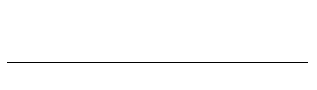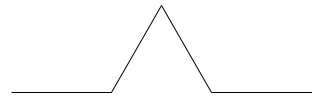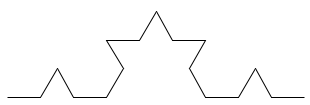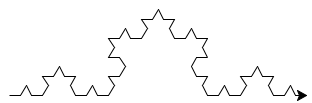# CS101 - HW3 Prelab

Complete as much of this prelab as possible before lab on Thursday / Friday. Ideally complete the entire prelab, but if you can get through Steps 4, 5, or 6 in the Koch curve problem that would be a good start.

From within the Thonny application, create a single Python file username_hw3.py with all your work for this assignment in it. Include a comment at the top with your name, the date and homework number, and your lab section.

### [7 points] Introducing the Turtle

This week we will learn one approach for doing some simple drawing in Python. We will use the `turtle` library. You can find more information in the turtle documentation. A quick reference is included at the bottom of this lab. Give it a perusal before you move on.

Here is a simple function that draws a square. Note that `length` is a parameter to the function so the function can draw squares of all sizes.

``````
def drawSquare(length):
"""
Draw a square with sides of size length.
"""
turtle.forward(length)
turtle.right(90)
turtle.forward(length)
turtle.right(90)
turtle.forward(length)
turtle.right(90)
turtle.forward(length)
turtle.right(90)

``````

To see this function in action, use this "helper" function:

``````
def drawSquareScene():
"""
Helper function to call drawSquare() function.
"""
drawSquare(100)
turtle.done()

``````

Paste both of these functions into a script. Begin your script with `import turtle`. Run the script and then call `drawSquareScene()` in the interpreter.

Once you have seen the turtle draw the square, close the turtle window.

Notice we used a function to abstract the turtle’s behavior. When you call `drawSquare()`, it draws a square and we no longer worry about the process. Also notice that we didn’t put anything into `drawSquare()` that wasn’t directly related to drawing the square. This means we can draw any size square, anywhere on the screen and in any color, provided we change those things before calling `drawSquare()`.

### [10 points] The Koch Curve

Write a recursive function ```drawKoch(length, generations)``` that draws a fractal shape called the Koch Curve. The Koch curve is a fractal – a shape that is self-similar, that is, it can be decomposed into smaller versions of the same shape.

Many of the techniques that we use to generate fractals computationally rely on the repetition of a set of steps, so we tend to talk about generations, which are the number of iterations that we used to produce the drawing. The Koch curve is a fairly simple one, and we can see its structure most clearly, by looking at how it changes from one generation to the next.

The starting place (generation 0) is a straight line.For the first generation, we cut the line into thirds and replace the middle segment with two segments of the same length, forming two sides of an equilateral triangle.The second generation is formed by applying this same process to every line in generation 1.Further generations are formed by repeating this process, taking each line, breaking it into thirds and replacing the middle segment with a bump. Conceptually, if you take this out to infinity, you will have a very curious mathematical phenomenon – an infinitely long line between two fixed points a finite distance away that also occupies a finite area. In practical terms, however, when the length of the line becomes 1 pixel long, that is pretty much as small as we can get.To actually draw the shape, our process will really be the opposite of what is described above. We don’t want to draw a line and then go back and remove a piece. Instead, we will start with the highest generation of the fractal first. If you think about a generation 2 curve, it is really made up of four generation 1 curves that are each 1/3 the length of the generation 2 curve. But each of those generation 1 curves are made up of generation 0 curves 1/3 of that length. What that means is that we don’t actually draw any of the higher generation curves, the only thing we draw is a whole bunch of generation 0 curves (straight lines!) in different locations.

#### Writing the function

• Step 1: Copy this function into your script. There is some boilerplate that just moves the turtle over a little to give us some more room. The only really important thing in there is the call to your `drawKoch()` function.
``````
def drawKochScene():
""" Setup the canvas for drawing the curve and draw it."""

# pick up the pen and move the turtle so it starts at the left edge of the canvas
turtle.penup()
turtle.goto(-turtle.window_width()/2 + 20,0)
turtle.pendown()

# draw the curve by calling your function
drawKoch(30, 0)

# finished
turtle.done()

``````
• Step 2: Start writing the `drawKoch()` function. Define the function and make sure it takes parameters `length` and `generations` (it is OK to abbreviate if you wish, eg, to shorter variable names such as `L` and `gen`). We will be drawing this shape using a recursive function. The `generations` parameter of the function will control the recursion. The base case is when `generations` is 0. In that case, the turtle should just draw a straight line of length `length` (it doesn’t matter which way the turtle is facing, we just go forward).

• Step 3: Write the base case. When `generations` is 0, draw a straight line of length `length`.

• Step 4: Test this by calling drawKochScene() from the interpreter. It should draw a line that is 30 pixels long.

• Step 5: We are going to start work on the recursive case, but it won’t be recursive yet. When `generations` is greater than 0, draw a first generation curve. We want the curve to fit within `length` pixels. So, move forward `length/3` pixels. Turn left by 60 degrees. Move forward `length/3` pixels (putting you at the apex of the triangle). Turn right and draw the second leg of the triangle. Turn left again and draw the final base line. You should now have a generation 1 curve that fits on a length `length` line

• Step 6: Test, and make sure that when you change the number of generations in `drawKochScene()` that you get a generation one curve.

• Step 7: Time to go recursive. Instead of drawing straight lines in the recursive case, each call to forward should be replaced with a call that draws a Koch curve of the same length (i.e., one third `length`), and one generation less.

• Step 8: Test this by entering some different generations into the call in `drawKochScene()`. We recommend starting small with something like a length of 200 and 1 generation just to make sure your code is working. Then you can work up to bigger curves and higher generations. IMPORTANT Keep the number of generations low or you will be waiting for it to finish forever. If you want to try higher generations, you should turn off the tracer (the tracer is what animates the drawing process. With it off, you just get the final picture). You can do that by putting the line `turtle.tracer(False)` before it draws and the line `turtle.update()` when you have something you want to display (i.e., before `turtle.done()`). A second problem you may encounter is if the length gets to be less than 1 before you hit the base case, the line segments drawn by the base case will all have a length of 0, and the curve will not draw at all.

### What should be in the file

For the prelab portion, you should have 4 functions (turn in with the rest of HW3): `drawSquare()`, `drawSquareScene()`, `drawKoch()`, `drawKochScene()`. Each function should have a docstring, and the top of the file should include a comment listing (at a minimum) your name, the date and homework number, and your lab section. You will continue working on this same Python file and add more function definitions when you continue working on the rest of Homework 3.

### Turtle reference

Python includes a library called `turtle`, which allows us to draw some simple graphics with a turtle that can draw wherever it walks. So, we issue commands to tell the turtle where to go, and a drawing appears as it moves. Here are the most important commands. More can be found in the online documentation.

`forward(distance)`
Move the turtle forward by the specified `distance` in the direction it is facing.
`backward(distance)`
Move the turtle backwards by the specified `distance` in the opposite direction to where it is facing.
`right(angle)`
Turn the turtle to its right by the specified `angle`. Note that this can be any angle, not the four cardinal directions we had with LightBot.
`left(angle)`
Turn the turtle to its left by the specified `angle`.
`goto(x, y)`
Drive the turtle immediately to the specified location without changing its orientation.
`pendown()`
Put the pen down so that the movement of the turtle leaves a track.
`penup()`
Pick the pen up so that there are no tracks when the turtle moves.
`pencolor(colorstring)`
Change the color of the pen that the turtle is using. This can be a string such as ‘red’, ‘yellow’, ‘blue’, etc. Of course, you have to pick a valid color name.
`fillcolor(colorstring)`
When this is set, the turtle will try to “fill in” any closed shapes that you draw with the given color.
`begin_fill()`
Call this before starting to draw a filled shape.
`end_fill()`
Call this function when you have finished drawing a shape that you want to be filled. This tells the turtle the shape is done and that it can try and fill it in.
`tracer(state)`
Turn on or off watching the turtle trace out the shapes. This is useful when you are trying to draw complex shapes and don’t want to wait. Pass in either `True` or `False` to turn the tracing on or off.
`update()`
If you turn off tracing, you should use this function to get everything the turtle has drawn so far to appear.
`window_width()`
Returns the current width of the turtle window.
`window_height()`
Returns the current height of the current turtle window.